Autocoders are a family of neural network models aiming to learn compressed latent variables of high-dimensional data. Starting from the basic autocoder model, this post reviews several variations, including denoising, sparse, and contractive autoencoders, and then Variational Autoencoder (VAE) and its modification beta-VAE.

Autocoder is invented to reconstruct high-dimensional data using a neural network model with a narrow bottleneck layer in the middle (oops, this is probably not true for Variational Autoencoder, and we will investigate it in details in later sections). A nice byproduct is dimension reduction: the bottleneck layer captures a compressed latent encoding. Such a low-dimensional representation can be used as en embedding vector in various applications (i.e. search), help data compression, or reveal the underlying data generative factors.

## Autoencoder

Autoencoder is a neural network designed to learn an identity function in an unsupervised way to reconstruct the original input while compressing the data in the process so as to discover a more efficient and compressed representation. The idea was originated in the 1980s, and later promoted by the seminal paper by Hinton & Salakhutdinov, 2016.

It consists of two networks:

• Encoder network: It translates the original high-dimension input into the latent low-dimensional code. The input size is larger than the output size.
• Decoder network: The decoder network recovers the data from the code, likely with larger and larger output layers.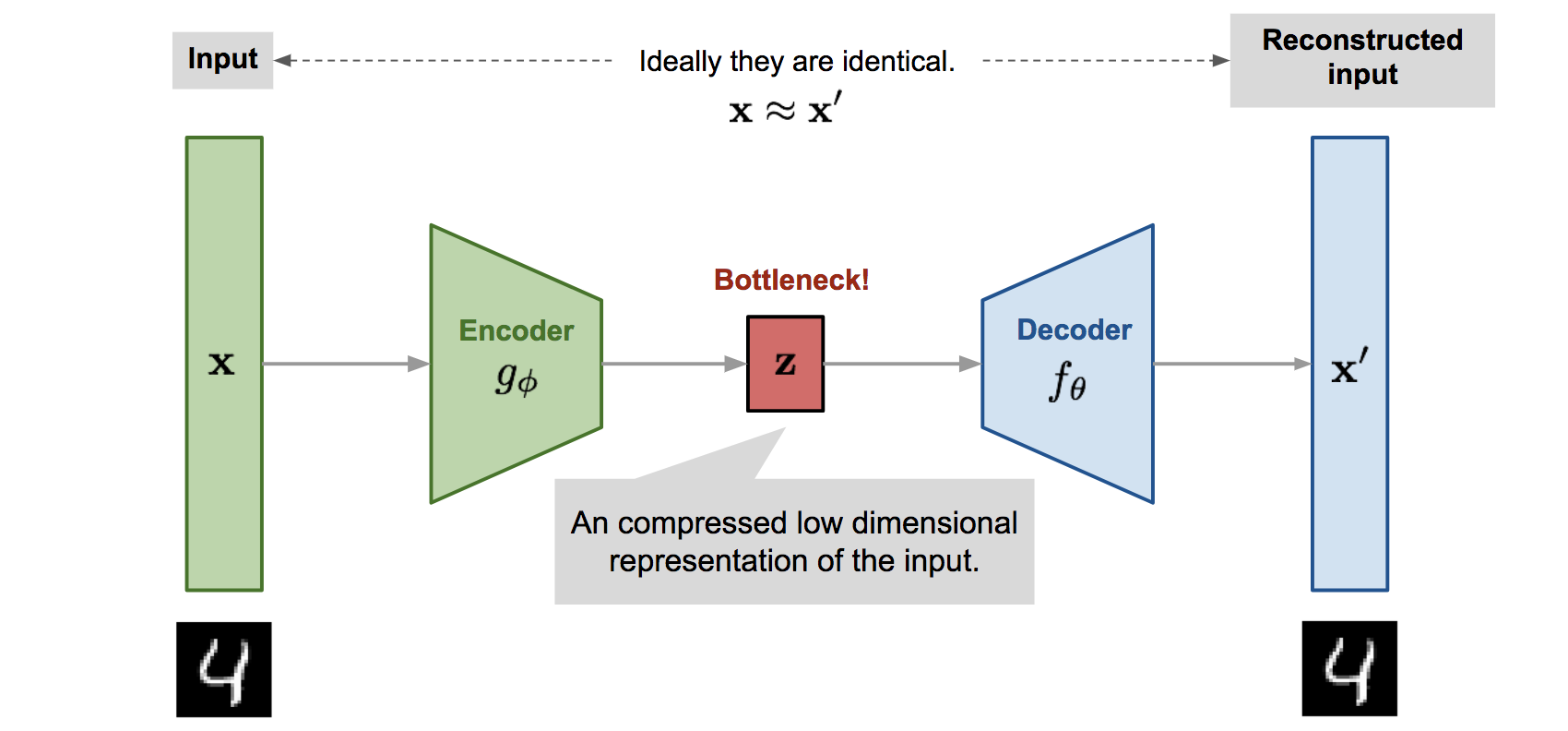Fig. 1. Illustration of autoencoder model architecture.

The encoder network essentially accomplishes the dimensionality reduction, just like how we would use Principal Component Analysis (PCA) or Matrix Factorization (MF) for. In addition, the autoencoder is explicitly optimized for the data reconstruction from the code. A good intermediate representation not only can capture latent variables, but also benefits a full decompression process.

The model contains an encoder function parameterized by and a decoder function parameterized by . The low-dimensional code learned for input in the bottleneck layer is and the reconstructed input is .

The parameters are learned together to output a reconstructed data sample same as the original input, , or in other words, to learn an identity function. There are various metrics to quantify the difference between two vectors, such as cross entropy when the activation function is sigmoid, or as simple as MSE loss:

## Denoising Autoencoder

Since the autoencoder learns the identity function, we are facing the risk of “overfitting” when there are more network parameters than the number of data points.

To avoid overfitting and improve the robustness, Denoising Autoencoder (Vincent et al. 2008) proposed a modification to the basic autoencoder. The input is partially corrupted by adding noises to or masking some values of the input vector in a stochastic manner, . Then the model is trained to recover the original input (Note: Not the corrupt one!).

where defines the mapping from the true data samples to the noisy or corrupted ones.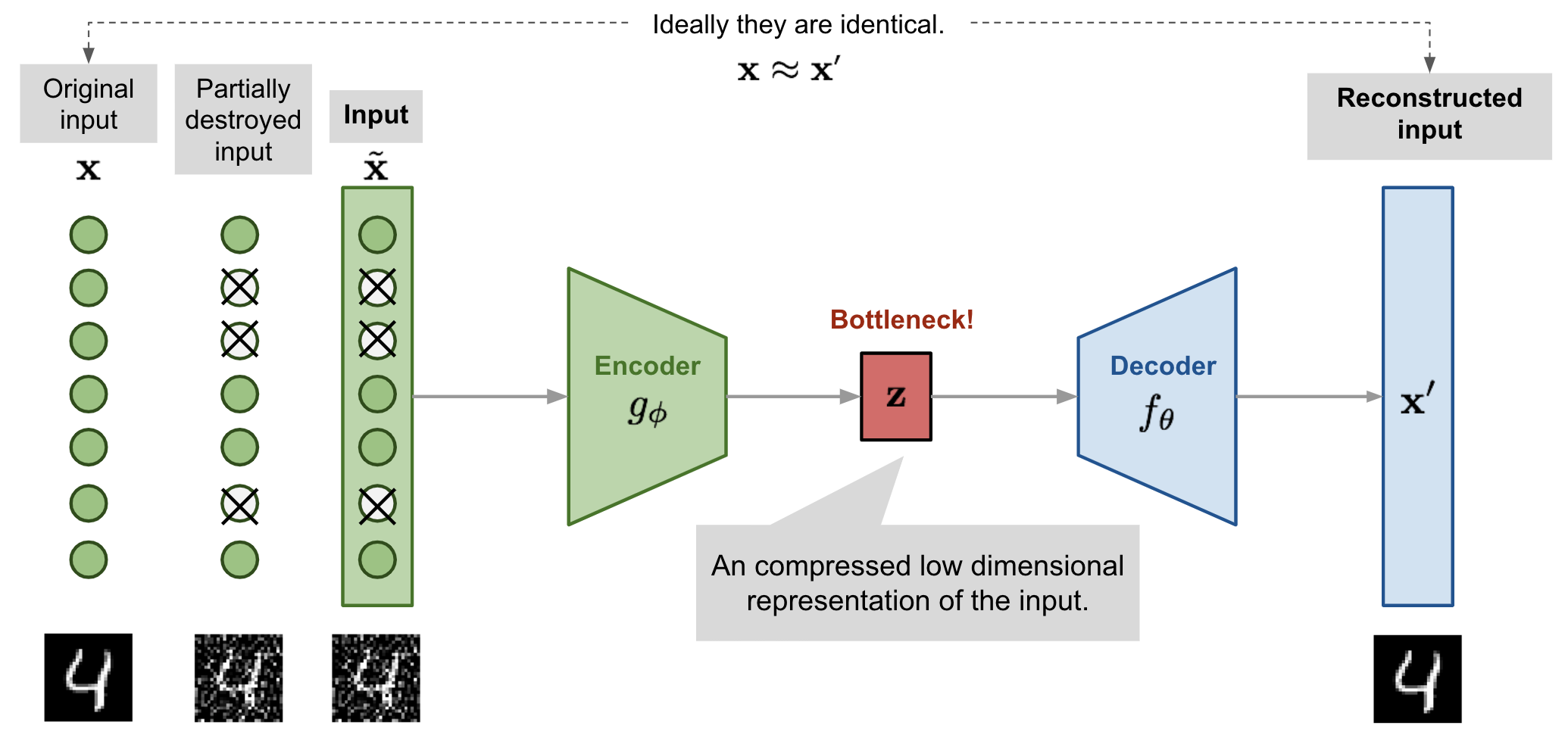Fig. 2. Illustration of denoising autoencoder model architecture.

This design is motivated by the fact that humans can easily recognize an object or a scene even the view is partially occluded or corrupted. To “repair” the partially destroyed input, the denoising autoencoder has to discover and capture relationship between dimensions of input in order to infer missing pieces.

For high dimensional input with high redundancy, like images, the model is likely to depend on evidence gathered from a combination of many input dimensions to recover the denoised version (sounds like the attention mechanism, right?) rather than to overfit one dimension. This builds up a good foundation for learning robust latent representation.

The noise is controlled by a stochastic mapping , and it is not specific to a particular type of corruption process (i.e. masking noise, Gaussian noise, salt-and-pepper noise, etc.). Naturally the corruption process can be equipped with prior knowledge

In the experiment of the original DAE paper, the noise is applied in this way: a fixed proportion of input dimensions are selected at random and their values are forced to 0. Sounds a lot like dropout, right? Well, the denoising autoencoder was proposed in 2008, 4 years before the dropout paper (Hinton, et al. 2012) ;)

## Sparse Autoencoder

Sparse Autoencoder applies a “sparse” constraint on the hidden unit activation to avoid overfitting and improve robustness. It forces the model to only have a small number of hidden units being activated at the same time, or in other words, one hidden neuron should be inactivate most of time.

Recall that common activation functions include sigmoid, tanh, relu, leaky relu, etc. A neuron is activated when the value is close to 1 and inactivate with a value close to 0.

Let’s say there are neurons in the -th hidden layer and the activation function for the -th neuron in this layer is labelled as , . The fraction of activation of this neuron is expected to be a small number , known as sparsity parameter; a common config is .

This constraint is achieved by adding a penalty term into the loss function. The KL-divergence measures the difference between two Bernoulli distributions, one with mean and the other with mean . The hyperparameter controls how strong the penalty we want to apply on the sparsity loss.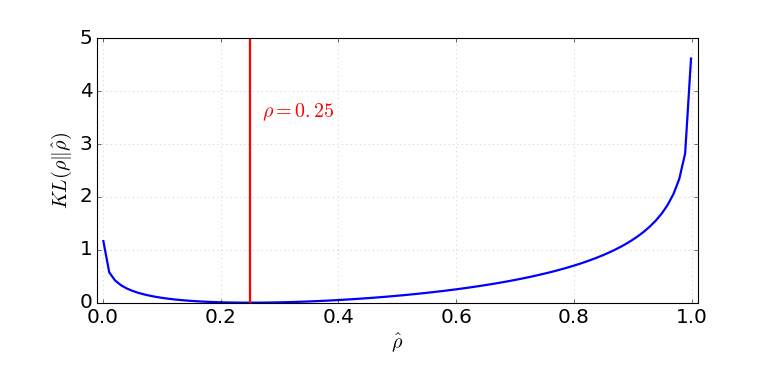Fig. 4. The KL divergence between a Bernoulli distribution with mean and a Bernoulli distribution with mean .

-Sparse Autoencoder

In -Sparse Autoencoder (Makhzani and Frey, 2013), the sparsity is enforced by only keeping the top k highest activations in the bottleneck layer with linear activation function. First we run feedforward through the encoder network to get the compressed code: . Sort the values in the code vector . Only the k largest values are kept while other neurons are set to 0. This can be done in a ReLU layer with an adjustable threshold too. Now we have a sparsified code: . Compute the output and the loss from the sparsified code, . And, the back-propagation only goes through the top k activated hidden units!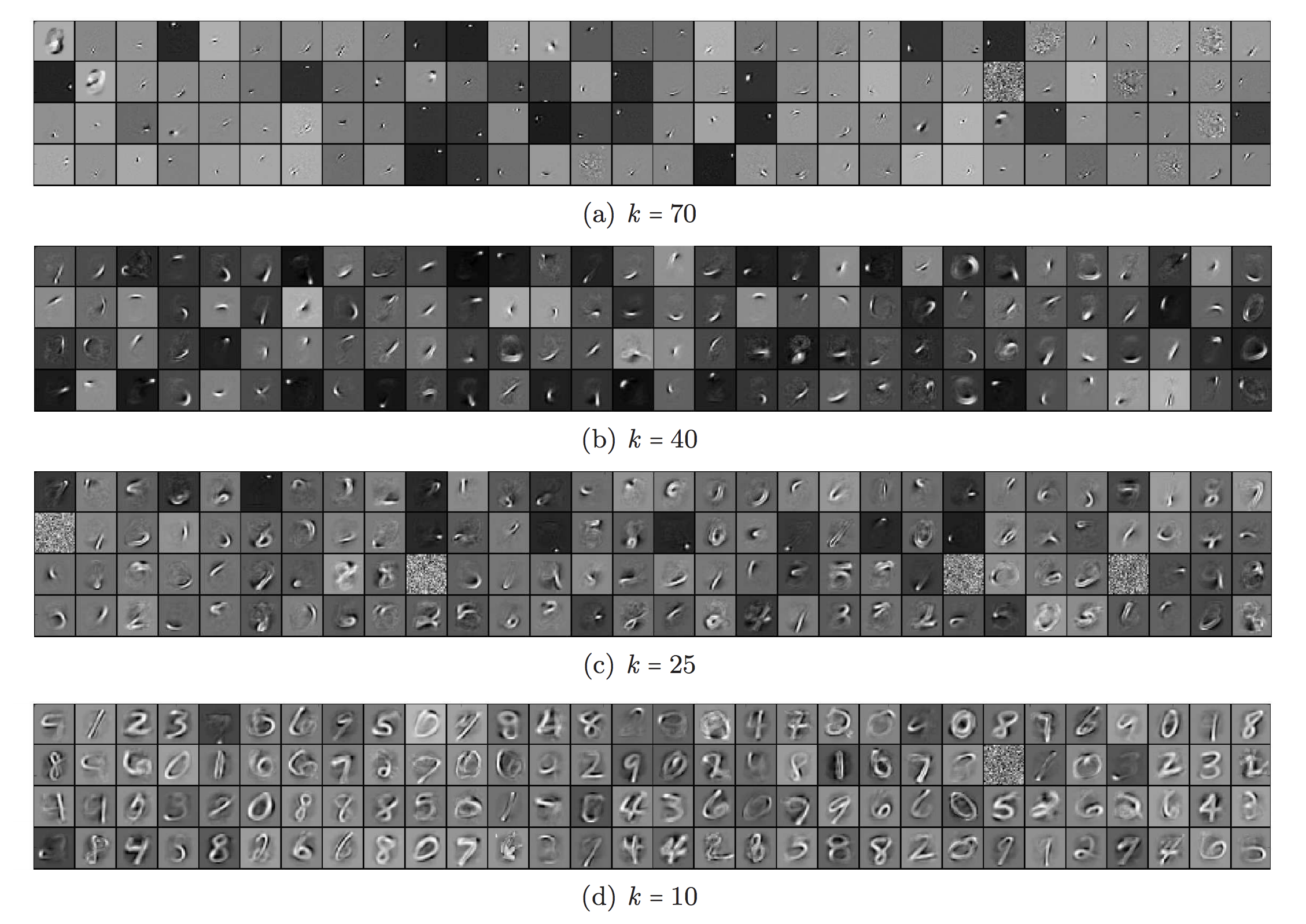Fig. 5. Filters of the k-sparse autoencoder for different sparsity levels k, learnt from MNIST with 1000 hidden units.. (Image source: Makhzani and Frey, 2013)

## Contractive Autoencoder

Similar to sparse autoencoder, Contractive Autoencoder (Rifai, et al, 2011) encourages the learned representation to stay in a contractive space for better robustness.

It adds a term in the loss function to penalize the representation being too sensitive to the input, and thus improve the robustness to small perturbations around the training data points. The sensitivity is measured by the Frobenius norm of the Jacobian matrix of the encoder activations with respect to the input:

where is one unit output in the compressed code .

This penalty term is the sum of squares of all partial derivatives of the learned encoding with respect to input dimensions. The authors claimed that empirically this penalty was found to carve a representation that corresponds to a lower-dimensional non-linear manifold, while staying more invariant to majority directions orthogonal to the manifold.

## VAE: Variational Autoencoder

The idea of Variational Autoencoder (Kingma & Welling, 2014), short for VAE, is actually less similar to all the autoencoder models above, but deeply rooted in the methods of variational bayesian and graphical model.

Instead of mapping the input into a fixed vector, we want to map it into a distribution. Let’s label this distribution as , parameterized by . The relationship between the data input and the latent encoding vector can be fully defined by:

• Prior
• Likelihood
• Posterior

Assuming that we know the real parameter for this distribution. In order to generate a sample that looks like a real data point , we follow these steps:

1. First, sample a from a prior distribution .
2. Then a value is generated from a conditional distribution .

The optimal parameter is the one that maximizes the probability of generating real data samples:

Commonly we use the log probabilities to convert the product on RHS to a sum:

Now let’s update the equation to better demonstrate the data generation process so as to involve the encoding vector:

Unfortunately it is not easy to compute in this way, as it is very expensive to check all the possible values of and sum them up. To narrow down the value space to facilitate faster search, we would like to introduce a new approximation function to output what is a likely code given an input , , parameterized by .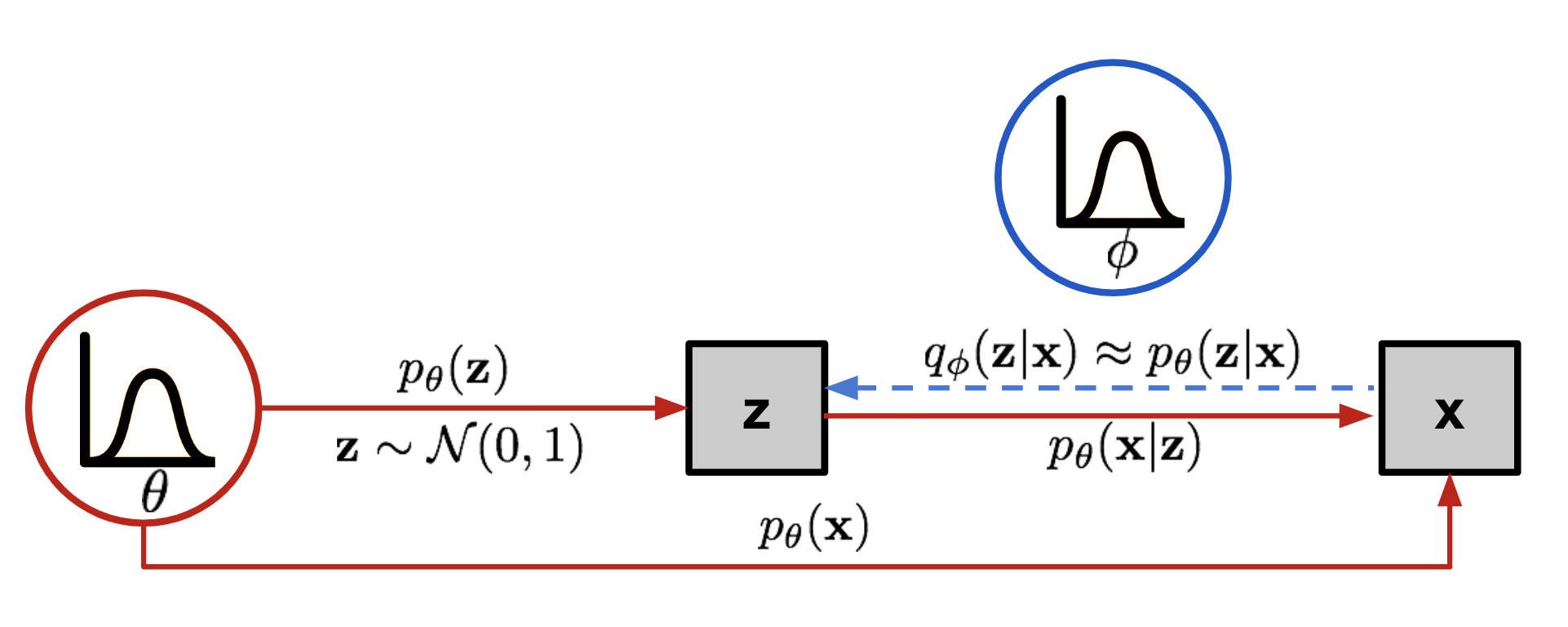Fig. 6. The graphical model involved in Variational Autoencoder. Solid lines denote the generative distribution and dashed lines denote the distribution to approximate the intractable posterior .

Now the structure looks a lot like an autoencoder:

• The conditional probability defines a generative model, similar to the decoder introduced above. is also known as probabilistic decoder.
• The approximation function is the probabilistic encoder, playing a similar role as above.

### Loss Function

The estimated posterior should be very close to the real one . We can use Kullback-Leibler divergence to quantify the distance between these two distributions. KL divergence measures how much information is lost if the distribution Y is used to represent X.

In our case we want to minimize with respect to .

But why use (reversed KL) instead of (forward KL)? Eric Jang has a great explanation in his post on Bayesian Variational methods. As a quick recap: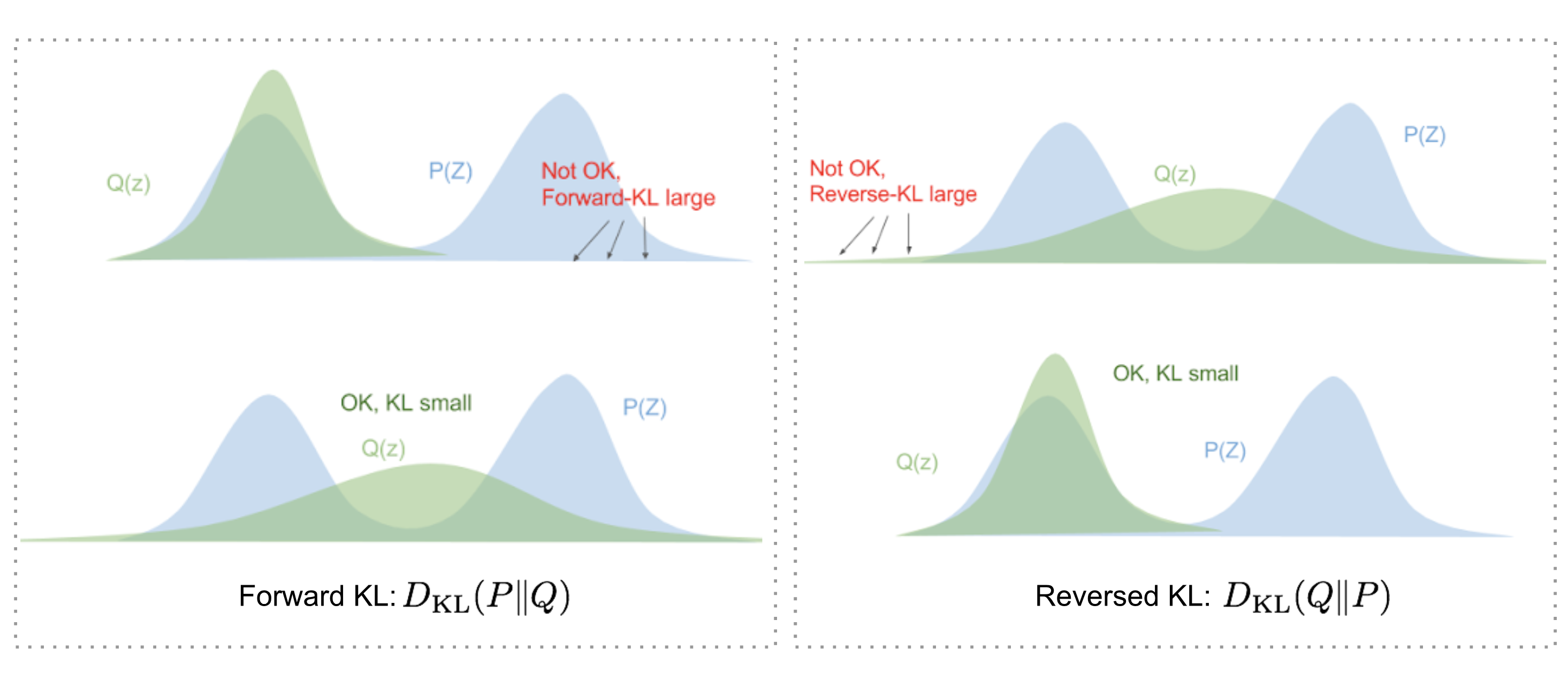Fig. 7. Forward and reversed KL divergence have different demands on how to match two distributions. (Image source: blog.evjang.com/2016/08/variational-bayes.html)

• Forward KL divergence: ; we have to ensure that Q(z)>0 wherever P(z)>0. The optimized variational distribution has to cover over the entire .
• Reversed KL divergence: ; minimizing the reversed KL divergence squeezes the under .

Let’s now expand the equation:

So we have:

Once rearrange the left and right hand side of the equation,

The LHS of the equation is exactly what we want to maximize when learning the true distributions: we want to maximize the (log-)likelihood of generating real data (that is ) and also minimize the difference between the real and estimated posterior distributions (the term works like a regularizer). Note that is fixed with respect to .

The negation of the above defines our loss function:

In Variational Bayesian methods, this loss function is known as the variational lower bound, or evidence lower bound. The “lower bound” part in the name comes from the fact that KL divergence is always non-negative and thus is the lower bound of .

Therefore by minimizing the loss, we are maximizing the lower bound of the probability of generating real data samples.

### Reparameterization Trick

The expectation term in the loss function invokes generating samples from . Sampling is a stochastic process and therefore we cannot backpropagate the gradient. To make it trainable, the reparameterization trick is introduced: It is often possible to express the random variable as a deterministic variable , where is an auxiliary independent random variable, and the transformation function parameterized by converts to .

For example, a common choice of the form of is a multivariate Gaussian with a diagonal covariance structure:

where refers to element-wise product.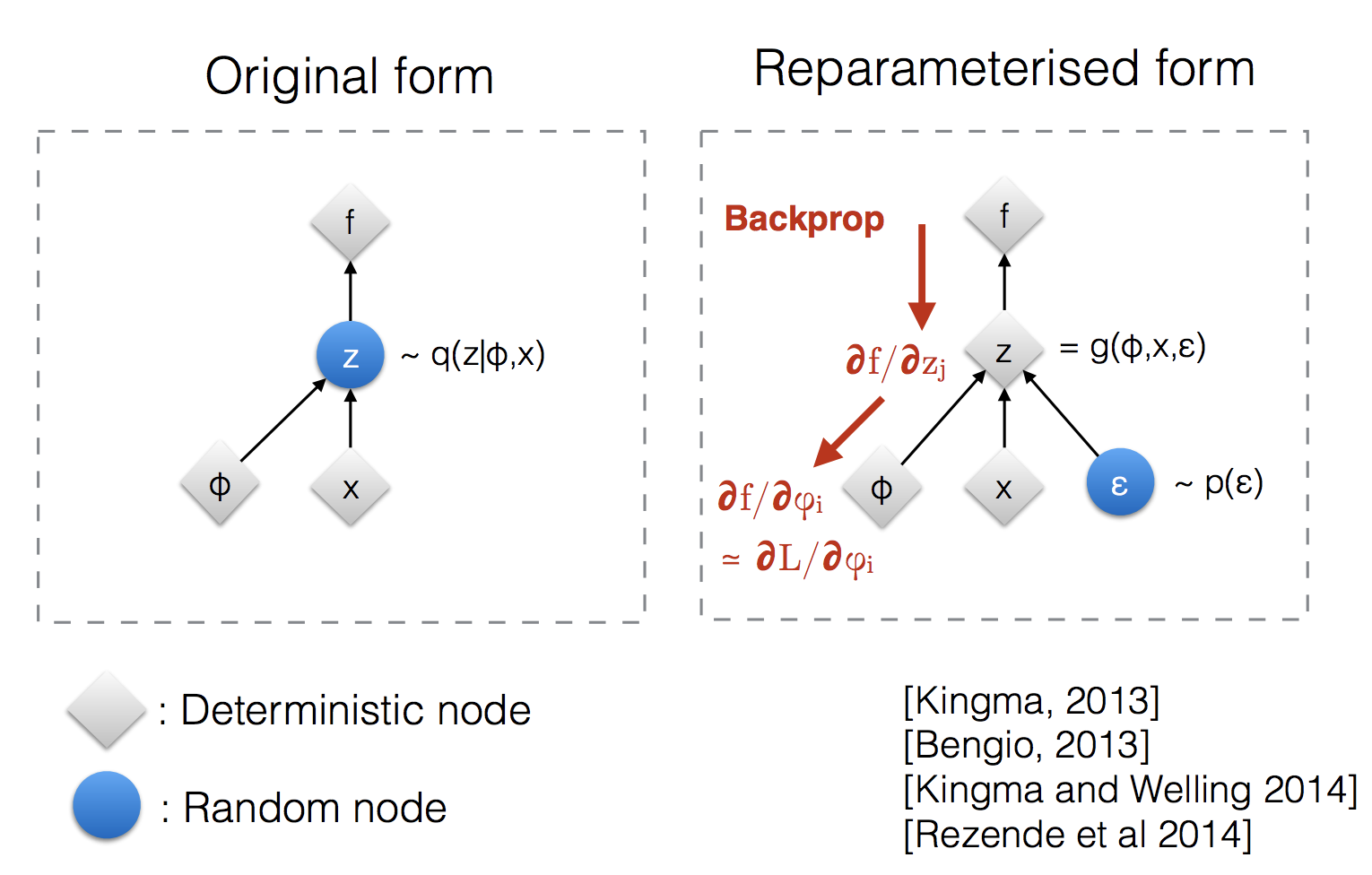Fig. 8. Illustration of how the reparameterization trick makes the sampling process trainable.(Image source: Slide 12 in Kingma’s NIPS 2015 workshop talk)

The reparameterization trick works for other types of distributions too, not only Gaussian. In the multivariate Gaussian case, we make the model trainable by learning the mean and variance of the distribution, and , explicitly using the reparameterization trick, while the stochasticity remains in the random variable .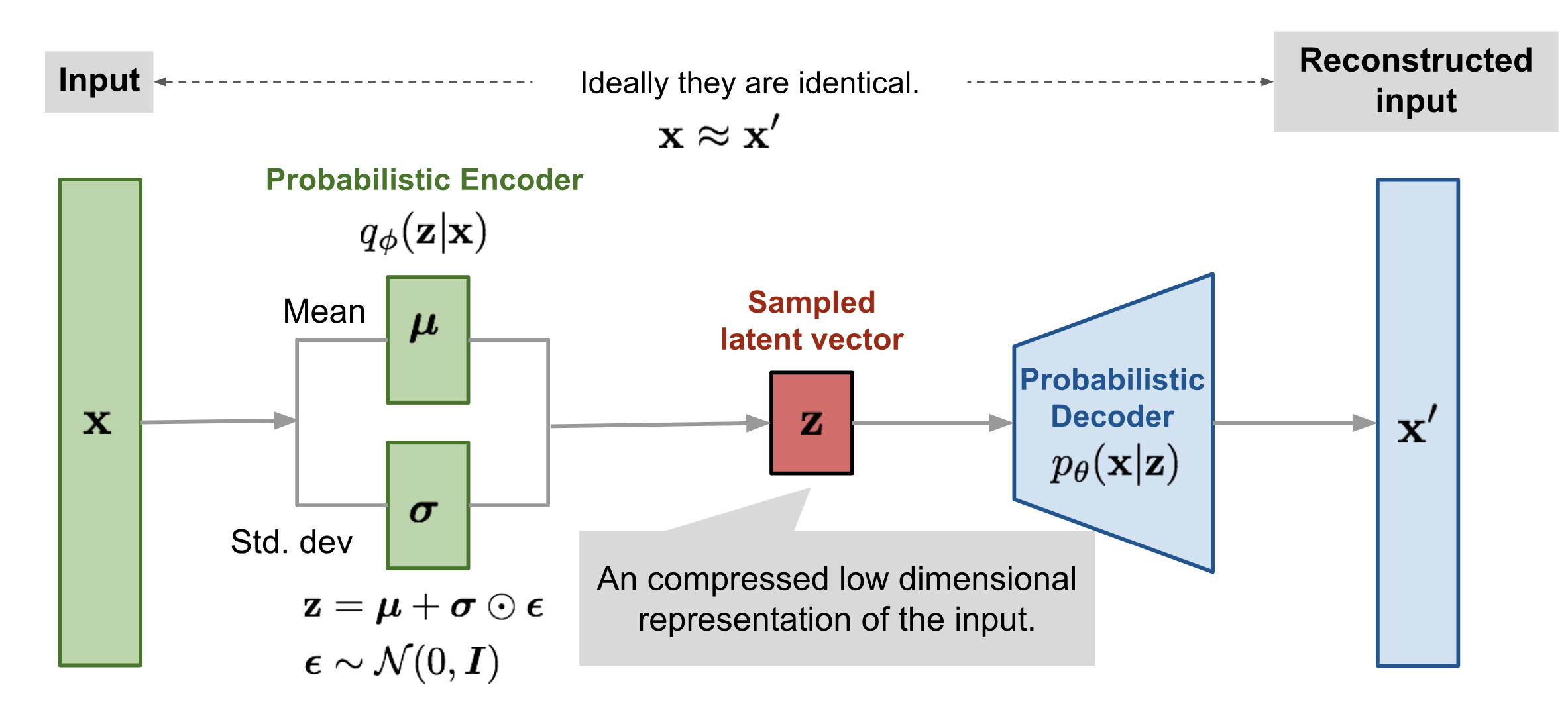Fig. 9. Illustration of variational autoencoder model with the multivariate Gaussian assumption.

## Beta-VAE

If each variable in the inferred latent representation is only sensitive to one single generative factor and relatively invariant to other factors, we will say this representation is disentangled or factorized. One benefit that often comes with disentangled representation is good interpretability and easy generalization to a variety of tasks.

For example, a model trained on photos of human faces might capture the gentle, skin color, hair color, hair length, emotion, whether wearing a pair of glasses and many other relatively independent factors in separate dimensions. Such a disentangled representation is very beneficial to facial image generation.

-VAE (Higgins et al., 2017) is a modification of Variational Autoencoder with a special emphasis to discover disentangled latent factors. Following the same incentive in VAE, we want to maximize the probability of generating real data, while keeping the distance between the real and estimated posterior distributions small (say, under a small constant ):

We can rewrite it as a Lagrangian with a Lagrangian multiplier under the KKT condition. The above optimization problem with only one inequality constraint is equivalent to maximizing the following equation :

The loss function of -VAE is defined as:

where the Lagrangian multiplier is considered as a hyperparameter.

Since the negation of is the lower bound of the Lagrangian . Minimizing the loss is equivalent to maximizing the Lagrangian and thus works for our initial optimization problem.

When , it is same as VAE. When , it applies a stronger constraint on the latent bottleneck and limits the representation capacity of . For some conditionally independent generative factors, keeping them disentangled is the most efficient representation. Therefore a higher encourages more efficient latent encoding and further encourages the disentanglement. Meanwhile, a higher may create a trade-off between reconstruction quality and the extent of disentanglement.

Burgess, et al. (2017) discussed the distentangling in -VAE in depth with an inspiration by the information bottleneck theory and further proposed a modification to -VAE to better control the encoding representation capacity.

## Applications

Although all the autoencoder models introduced in this post work in an unsupervised fashion, they can be used for supervised applications too.

 Geoffrey E. Hinton, and Ruslan R. Salakhutdinov. “Reducing the dimensionality of data with neural networks.” Science 313.5786 (2006): 504-507.

 Pascal Vincent, et al. “Extracting and composing robust features with denoising autoencoders.” ICML, 2008.

 Pascal Vincent, et al. “Stacked denoising autoencoders: Learning useful representations in a deep network with a local denoising criterion.”. Journal of machine learning research 11.Dec (2010): 3371-3408.

 Geoffrey E. Hinton, Nitish Srivastava, Alex Krizhevsky, Ilya Sutskever, and Ruslan R. Salakhutdinov. “Improving neural networks by preventing co-adaptation of feature detectors.” arXiv preprint arXiv:1207.0580 (2012).

 Sparse Autoencoder by Andrew Ng.

 Alireza Makhzani, Brendan Frey (2013). “k-sparse autoencoder”. ICLR 2014.

 Salah Rifai, et al. “Contractive auto-encoders: Explicit invariance during feature extraction.” ICML, 2011.

 Diederik P. Kingma, and Max Welling. “Auto-encoding variational bayes.” ICLR 2014.

 Tutorial - What is a variational autoencoder? on jaan.io

 Youtube tutorial: Variational Autoencoders by Arxiv Insights

 Carl Doersch. “Tutorial on variational autoencoders.” arXiv:1606.05908, 2016.

 Irina Higgins, et al. ”-VAE: Learning basic visual concepts with a constrained variational framework.” ICLR 2017.

 Christopher P. Burgess, et al. “Understanding disentangling in beta-VAE.” NIPS 2017.